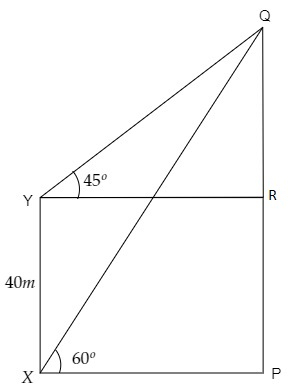# The angle of elevation of the top of a vertical tower $P Q$ from a point $X$ on the ground is $60^{\circ}$. At a point $Y, 40$ m vertically above $X$, the angle of elevation of the top is $45^{\circ}$. Calculate the height of the tower.

Given:

The angle of elevation of the top of a vertical tower $P Q$ from a point $X$ on the ground is $60^{\circ}$.

At a point $Y, 40$ m vertically above $X$, the angle of elevation of the top is $45^{\circ}$.

To do:

We have to calculate the height of the tower.

Solution:From the figure,

$\mathrm{XY}=40 \mathrm{~m}, \angle \mathrm{QXP}=60^{\circ}, \angle \mathrm{QYR}=45^{\circ}$

Let the height of the tower be $\mathrm{PQ}=h \mathrm{~m}$ and the distance between the tower and point $X$ be $\mathrm{XP}=x \mathrm{~m}$.

This implies,

$\mathrm{YR}=x \mathrm{~m}$

$\mathrm{PR}=40 \mathrm{~m}$

$\mathrm{QR}=h-40 \mathrm{~m}$

We know that,

$\tan \theta=\frac{\text { Opposite }}{\text { Adjacent }}$

$=\frac{\text { QR }}{YR}$

$\Rightarrow \tan 45^{\circ}=\frac{h-40}{x}$

$\Rightarrow 1=\frac{h-40}{x}$

$\Rightarrow x=h-40 \mathrm{~m}$...........(i)

Similarly,

$\tan \theta=\frac{\text { Opposite }}{\text { Adjacent }}$

$=\frac{\text { PQ }}{XP}$

$\Rightarrow \tan 60^{\circ}=\frac{h}{x}$

$\Rightarrow \sqrt3=\frac{h}{h-40}$                 [From (i)]

$\Rightarrow (h-40)\sqrt3=h \mathrm{~m}$

$\Rightarrow h(\sqrt3-1)=40\sqrt3 \mathrm{~m}$

$\Rightarrow h=\frac{40(1.732)}{1.732-1} \mathrm{~m}$

$\Rightarrow h=\frac{69.28}{0.732} \mathrm{~m}$

$\Rightarrow h=94.64 \mathrm{~m}$

Therefore, the height of the tower is $94.64 \mathrm{~m}$.

Updated on: 10-Oct-2022

72 Views